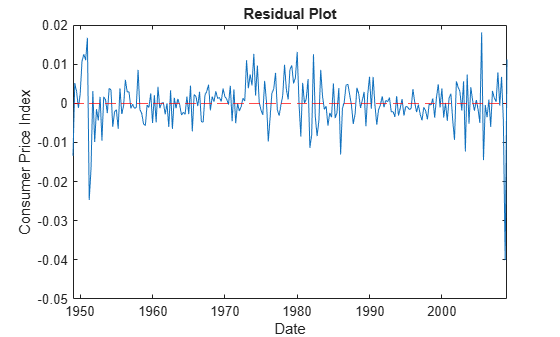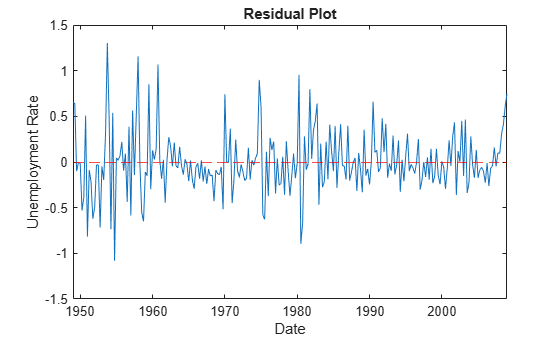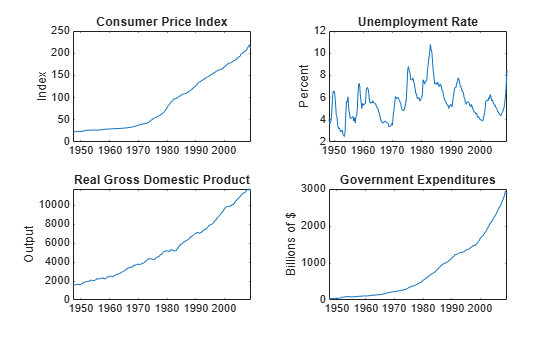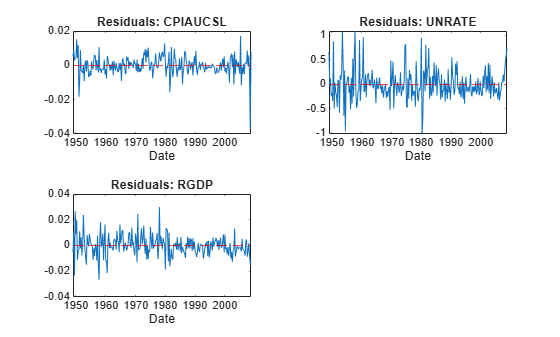# infer

Infer vector autoregression model (VAR) innovations

## Syntax

``E = infer(Mdl,Y)``
``E = infer(Mdl,Y,Name,Value)``
``````[E,logL] = infer(___)``````

## Description

example

``E = infer(Mdl,Y)` returns the inferred multivariate innovations series from evaluating the fully specified VAR(p) model `Mdl` at the response data `Y`.`

example

````E = infer(Mdl,Y,Name,Value)` uses additional options specified by one or more name-value arguments. For example, you can specify presample responses or exogenous predictor data.```

example

``````[E,logL] = infer(___)``` returns the loglikelihood objective function value evaluated at `E` using any of the input arguments in the previous syntaxes.```

## Examples

collapse all

Fit a VAR(4) model to the consumer price index (CPI) and unemployment rate data. Then, infer the model innovations using the estimated model.

Load the `Data_USEconModel` data set.

`load Data_USEconModel`

Plot the two series on separate plots.

```figure; plot(DataTable.Time,DataTable.CPIAUCSL); title('Consumer Price Index'); ylabel('Index'); xlabel('Date');``````figure; plot(DataTable.Time,DataTable.UNRATE); title('Unemployment Rate'); ylabel('Percent'); xlabel('Date');```Stabilize the CPI by converting it to a series of growth rates. Synchronize the two series by removing the first observation from the unemployment rate series.

```rcpi = price2ret(DataTable.CPIAUCSL); unrate = DataTable.UNRATE(2:end);```

Create a default VAR(4) model using the shorthand syntax.

`Mdl = varm(2,4);`

Estimate the model using the entire data set.

`EstMdl = estimate(Mdl,[rcpi unrate]);`

`EstMdl` is a fully specified, estimated `varm` model object.

Infer innovations from the estimated model.

`E = infer(EstMdl,[rcpi unrate]);`

`E` is a 241-by-2 matrix of inferred innovations. The first and second columns contain the residuals corresponding to the CPI growth rate and unemployment rate, respectively.

Alternatively, you can return residuals when you call `estimate` by supplying an output variable in the fourth position.

Plot the residuals on separate plots. Synchronize the residuals with the dates by removing any missing observations from the data and removing the first `Mdl.P` dates.

```idx = all(~isnan([rcpi unrate]),2); datesr = DataTable.Time(idx); figure; plot(datesr((Mdl.P + 1):end),E(:,1)); ylabel('Consumer price index'); xlabel('Date'); title('Residual plot'); hold on plot([min(datesr) max(datesr)],[0 0],'r--'); hold off``````figure; plot(datesr((Mdl.P + 1):end),E(:,2)); ylabel('Unemployment rate'); xlabel('Date'); title('Residual plot'); hold on plot([min(datesr) max(datesr)],[0 0],'r--'); hold off```The residuals corresponding to the CPI growth rate exhibit heteroscedasticity because the series appears to cycle through periods of higher and lower variance.

Estimate a VAR(4) model of the consumer price index (CPI), the unemployment rate, and the gross domestic product (GDP). Include a linear regression component containing the current quarter and the last four quarters of government consumption expenditures and investment (GCE). Infer model innovations.

Load the `Data_USEconModel` data set. Compute the real GDP.

```load Data_USEconModel DataTable.RGDP = DataTable.GDP./DataTable.GDPDEF*100;```

Plot all variables on separate plots.

```figure; subplot(2,2,1) plot(DataTable.Time,DataTable.CPIAUCSL); ylabel('Index'); title('Consumer Price Index'); subplot(2,2,2) plot(DataTable.Time,DataTable.UNRATE); ylabel('Percent'); title('Unemployment Rate'); subplot(2,2,3) plot(DataTable.Time,DataTable.RGDP); ylabel('Output'); title('Real Gross Domestic Product'); subplot(2,2,4) plot(DataTable.Time,DataTable.GCE); ylabel('Billions of \$'); title('Government Expenditures');```Stabilize the CPI, GDP, and GCE by converting each to a series of growth rates. Synchronize the unemployment rate series with the others by removing its first observation.

```inputVariables = {'CPIAUCSL' 'RGDP' 'GCE'}; Data = varfun(@price2ret,DataTable,'InputVariables',inputVariables); Data.Properties.VariableNames = inputVariables; Data.UNRATE = DataTable.UNRATE(2:end);```

Expand the GCE rate series to a matrix that includes its current value and up through four lagged values. Remove the `GCE` variable from `Data`.

```rgcelag4 = lagmatrix(Data.GCE,0:4); Data.GCE = [];```

Create a default VAR(4) model using the shorthand syntax.

```Mdl = varm(3,4); Mdl.SeriesNames = ["rcpi" "unrate" "rgdpg"];```

Estimate the model using the entire sample. Specify the GCE matrix as data for the regression component.

`EstMdl = estimate(Mdl,Data.Variables,'X',rgcelag4);`

Infer innovations from the estimated model. Supply the predictor data. Return the loglikelihood objective function value.

```[E,logL] = infer(EstMdl,Data.Variables,'X',rgcelag4); logL```
```logL = 1.7056e+03 ```

`E` is a 240-by-3 matrix of inferred innovations. The columns contain the residuals corresponding to the CPI growth rate, unemployment rate, and GDP growth rate, respectively.

Plot the residuals on separate plots. Synchronize the residuals with the dates by removing any missing observations from the data and removing the first `Mdl.P` dates.

```idx = all(~isnan([Data.Variables rgcelag4]),2); datesr = DataTable.Time(idx); figure; for j = 1:Mdl.NumSeries subplot(2,2,j) plot(datesr((Mdl.P + 1):end),E(:,j)); ylabel(Mdl.SeriesNames{j}); xlabel('Date'); title('Residual plot'); hold on plot([min(datesr) max(datesr)],[0 0],'r--'); hold off end```The residuals corresponding to the CPI and GDP growth rates exhibit heteroscedasticity because the CPI series appears to cycle through periods of higher and lower variance. Also, the first half of the GDP series seems to have higher variance than the latter half.

## Input Arguments

collapse all

VAR model, specified as a `varm` model object created by `varm` or `estimate`. `Mdl` must be fully specified.

Response data, specified as a `numobs`-by-`numseries` numeric matrix or a `numobs`-by-`numseries`-by-`numpaths` numeric array.

`numobs` is the sample size. `numseries` is the number of response series (`Mdl.NumSeries`). `numpaths` is the number of response paths.

Rows correspond to observations, and the last row contains the latest observation. `Y` represents the continuation of the presample response series in `Y0`.

Columns must correspond to the response variable names in `Mdl.SeriesNames`.

Pages correspond to separate, independent `numseries`-dimensional paths. Among all pages, responses in a particular row occur at the same time.

Data Types: `double`

### Name-Value Arguments

Specify optional pairs of arguments as `Name1=Value1,...,NameN=ValueN`, where `Name` is the argument name and `Value` is the corresponding value. Name-value arguments must appear after other arguments, but the order of the pairs does not matter.

Before R2021a, use commas to separate each name and value, and enclose `Name` in quotes.

Example: `'Y0',Y0,'X',X` uses the matrix `Y0` as presample responses and the matrix `X` as predictor data in the regression component.

Presample responses providing initial values for the model, specified as the comma-separated pair consisting of `'Y0'` and a `numpreobs`-by-`numseries` numeric matrix or a `numpreobs`-by-`numseries`-by-`numprepaths` numeric array.

`numpreobs` is the number of presample observations. `numprepaths` is the number of presample response paths.

Rows correspond to presample observations, and the last row contains the latest presample observation. `Y0` must have at least `Mdl.P` rows. If you supply more rows than necessary, `infer` uses only the latest `Mdl.P` observations.

Columns must correspond to the columns of `Y`.

Pages correspond to separate independent paths.

• If `Y0` is a matrix, then `infer` applies it to each path (page) in `Y`. Therefore, all paths in `Y` derive from common initial conditions.

• Otherwise, `infer` applies `Y0(:,:,j)` to `Y(:,:,j)`. `Y0` must have at least `numpaths` pages, and `infer` uses only the first `numpaths` pages.

Among all pages, observations in a particular row occur at the same time.

By default, `infer` uses `Y(1:Mdl.P,:)` as presample observations. This action reduces the effective sample size.

Data Types: `double`

Predictor data for the regression component in the model, specified as the comma-separated pair consisting of `'X'` and a numeric matrix containing `numpreds` columns.

`numpreds` is the number of predictor variables (`size(Mdl.Beta,2)`).

Rows correspond to observations, and the last row contains the latest observation. `infer` does not use the regression component in the presample period. Therefore, `X` must have at least as many observations as are used after the presample period.

• If you specify `Y0`, then `X` must have at least `numobs` rows (see `Y`).

• Otherwise, `X` must have at least `numobs``Mdl.P` observations to account for the presample removal.

In either case, if you supply more rows than necessary, `infer` uses the latest observations only.

Columns correspond to individual predictor variables. All predictor variables are present in the regression component of each response equation.

`infer` applies `X` to each path (page) in `Y`; that is, `X` represents one path of observed predictors.

By default, `infer` excludes the regression component, regardless of its presence in `Mdl`.

Data Types: `double`

Note

`NaN` values in `Y`, `Y0`, and `X` indicate missing values. `infer` removes missing values from the data by list-wise deletion.

1. If `Y` is a 3-D array, then `infer` horizontally concatenates the pages of `Y` to form a `numobs`-by-`(numpaths*numseries + numpreds)` matrix.

2. If a regression component is present, then `infer` horizontally concatenates `X` to `Y` to form a `numobs`-by-`numpaths*numseries + 1` matrix. `infer` assumes that the last rows of each series occur at the same time.

3. `infer` removes any row that contains at least one `NaN` from the concatenated data.

4. `infer` applies steps 1 and 3 to the presample paths in `Y0`.

This process ensures that the inferred output innovations of each path are the same size and are based on the same observation times. In the case of missing observations, the results obtained from multiple paths of `Y` can differ from the results obtained from each path individually.

This type of data reduction reduces the effective sample size.

## Output Arguments

collapse all

Inferred multivariate innovations series, returned as either a numeric matrix, or as a numeric array that contains columns and pages corresponding to `Y`.

• If you specify `Y0`, then `E` has `numobs` rows (see `Y`).

• Otherwise, `E` has `numobs``Mdl.P` rows to account for the presample removal.

Loglikelihood objective function value associated with the VAR model `Mdl`, returned as a numeric scalar or a `numpaths`-element numeric vector. `logL(j)` corresponds to the response path in `Y(:,:,j)`.

## Algorithms

• `infer` infers innovations by evaluating the VAR model `Mdl` with respect to the innovations using the supplied data `Y`, `Y0`, and `X`. The inferred innovations are

`${\stackrel{^}{\epsilon }}_{t}=\stackrel{^}{\Phi }\left(L\right){y}_{t}-\stackrel{^}{c}-\stackrel{^}{\beta }{x}_{t}-\stackrel{^}{\delta }t.$`

• `infer` uses this process to determine the time origin t0 of models that include linear time trends.

• If you do not specify `Y0`, then t0 = 0.

• Otherwise, `infer` sets t0 to `size(Y0,1)``Mdl.P`. Therefore, the times in the trend component are t = t0 + 1, t0 + 2,..., t0 + `numobs`, where `numobs` is the effective sample size (`size(Y,1)` after `infer` removes missing values). This convention is consistent with the default behavior of model estimation in which `estimate` removes the first `Mdl.P` responses, reducing the effective sample size. Although `infer` explicitly uses the first `Mdl.P` presample responses in `Y0` to initialize the model, the total number of observations in `Y0` and `Y` (excluding missing values) determines t0.

 Hamilton, James D. Time Series Analysis. Princeton, NJ: Princeton University Press, 1994.

 Johansen, S. Likelihood-Based Inference in Cointegrated Vector Autoregressive Models. Oxford: Oxford University Press, 1995.

 Juselius, K. The Cointegrated VAR Model. Oxford: Oxford University Press, 2006.

 Lütkepohl, H. New Introduction to Multiple Time Series Analysis. Berlin: Springer, 2005.